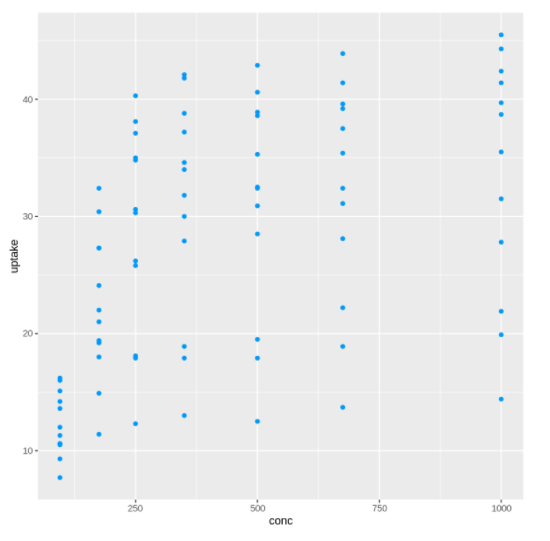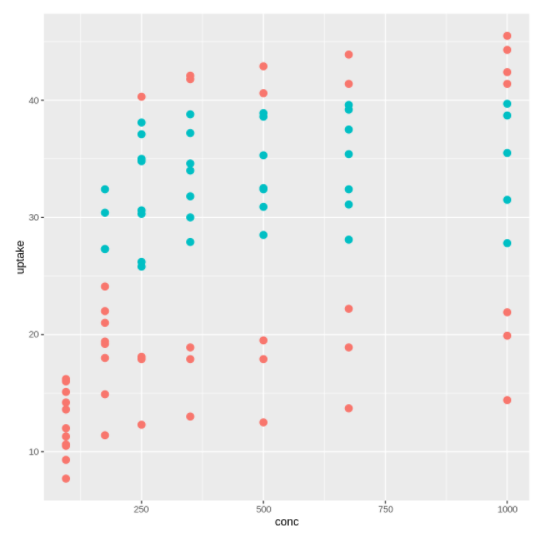# Learn Scatter Plot in R using ggplot2 with ExamplesOut:

Out:### Example 3: Scatter Plot Size in ggplot2

Out:

The geom_point function allows coloring the points based on some conditions. In the below examples the color of the points are set based on the range uptake variable of the CO2 dataset.

In :

```ggplot(CO2, mapping = aes(x = conc, y =uptake)) +
geom_point(size=3, aes(colour = uptake > 25 & uptake < 40), show.legend = FALSE)```

Out:The shapes of the scatter plot in ggplot2 can be changed quite easily with the shape argument in geom_point(). The various shapes have got a specific number that you need to pass to geom_point() for your needs. The various shapes and their corresponding number are shown below –